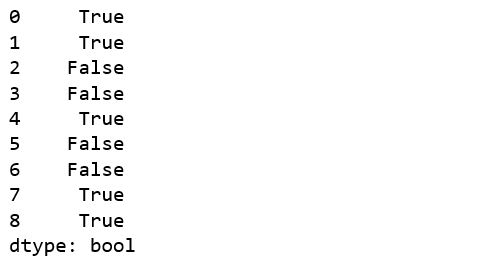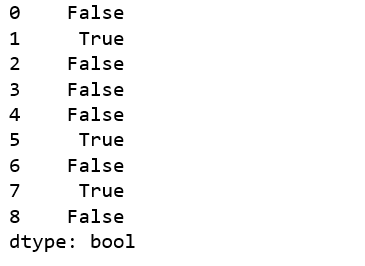Related Articles

# Python | Pandas Series.eq()

• Last Updated : 15 Oct, 2018

Python is a great language for doing data analysis, primarily because of the fantastic ecosystem of data-centric Python packages. Pandas is one of those packages and makes importing and analyzing data much easier.

Pandas` series.eq()` is used to compare every element of Caller series with passed series. It returns True for every element which is Equal to the element in passed series.

Note: The results are returned on the basis of comparison caller series = other series.

Syntax: Series.eq(other, level=None, fill_value=None)

Parameters:
other: other series to be compared with
level: int or name of level in case of multi level
fill_value: Value to be replaced instead of NaN

Return type: Boolean series

Example #1: Handling Null Values

In this example, two series are created using `pd.Series()`. The series contains some Null values and some equal values at same indices too. The series are compared using `.eq()` method and 5 is passed to fill_value parameter to replace NaN values by 5.

 `# importing pandas module  ``import` `pandas as pd  ``    ` `# importing numpy module ``import` `numpy as np ``    ` `# creating series 1 ``series1 ``=` `pd.Series([``70``, ``5``, ``0``, ``225``, ``1``, ``16``, np.nan, ``10``, np.nan]) ``    ` `# creating series 2 ``series2 ``=` `pd.Series([``70``, np.nan, ``2``, ``23``, ``1``, ``95``, ``53``, ``10``, ``5``]) ``  ` `# NaN replacement``replace_nan ``=` `5``  ` `# calling and returning to result variable``result ``=` `series1.eq(series2, fill_value ``=` `replace_nan)``  ` `# display ``result  `

Output:
As shown in output, True was returned wherever value in caller series was Equal to value in passed series. Also it can be seen Null values were replaced by 5 and the comparison was made using that value.Example #2: Calling on Series with str objects
In this example, two series are created using pd.Series(). The series contains some string values too. In case of strings, the comparison is made with their ASCII values.

 `# importing pandas module  ``import` `pandas as pd  ``   ` `# importing numpy module ``import` `numpy as np ``   ` `# creating series 1 ``series1 ``=` `pd.Series([``'Aaa'``, ``10``, ``'cat'``, ``43``, ``9``, ``'Dog'``, np.nan, ``'x'``, np.nan]) ``   ` `# creating series 2 ``series2 ``=` `pd.Series([``'vaa'``, np.nan, ``'Cat'``, ``23``, ``5``, ``'Dog'``, ``54``, ``'x'``, np.nan]) `` ` `# NaN replacement``replace_nan ``=` `10`` ` `# calling and returning to result variable``result ``=` `series1.eq(series2, fill_value ``=` `replace_nan)`` ` `# display ``result `

Output:
As it can be seen in output, in case of strings, the comparison was made using their ASCII values. True was returned wherever the string in Caller series was equal to string in passed series.Attention geek! Strengthen your foundations with the Python Programming Foundation Course and learn the basics.

To begin with, your interview preparations Enhance your Data Structures concepts with the Python DS Course. And to begin with your Machine Learning Journey, join the Machine Learning – Basic Level Course

My Personal Notes arrow_drop_up